Students can download Maths Chapter 2 Numbers and Sequences Ex 2.8 Questions and Answers, Notes, Samacheer Kalvi 10th Maths Guide Pdf helps you to revise the complete Tamilnadu State Board New Syllabus, helps students complete homework assignments and to score high marks in board exams.

## Tamilnadu Samacheer Kalvi 10th Maths Solutions Chapter 2 Numbers and Sequences Ex 2.8

Question 1.
Find the sum of first n terms of the G.P.
(i) 5, -3, $$\frac { 9 }{ 5 }$$,-$$\frac { 27 }{ 25 }$$, ……
(ii) 256,64,16,…….
(i) 5,-3,$$\frac { 9 }{ 5 }$$,$$\frac { 27 }{ 55 }$$, ….. n terms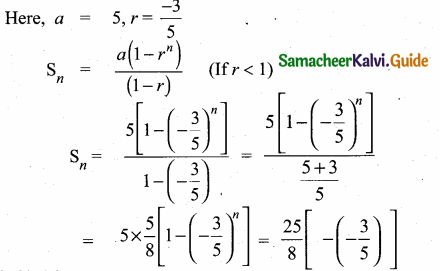(ii) 256,64,16,…….
Here a = 256, r = $$\frac { 64 }{ 256 }$$ = $$\frac { 1 }{ 4 }$$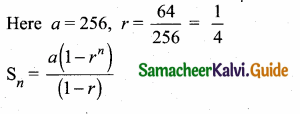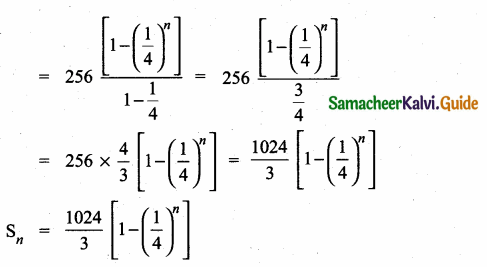Question 2.
Find the sum of first six terms of the G.P. 5,15,45,…
Here a = 5, r = $$\frac { 15 }{ 3 }$$ = 3, n = 6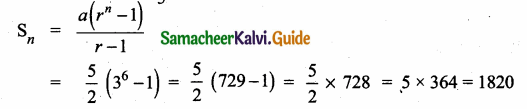Sum of first 6 terms = 1820

Question 3.
Find the first term of the G.P. whose common ratio 5 and whose sum to first 6 terms is 46872.
Common ratio (r) = 5
S6 = 46872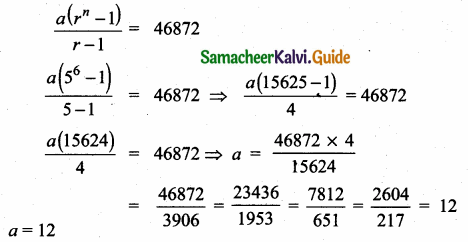The first term of the G.P. is 12.Question 4.
Find the sum to infinity of (i) 9 + 3 + 1 + ….(ii) 21 + 14 + $$\frac { 28 }{ 3 }$$ ……
(i) 9 + 3 + 1 + ….
a = 9, r = $$\frac { 3 }{ 9 }$$ = $$\frac { 1 }{ 3 }$$
Sum of infinity term = $$\frac { a }{ 1 – r }$$ = $$\frac{9}{1-\frac{1}{3}}$$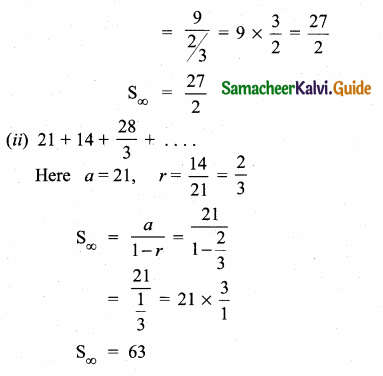Question 5.
If the first term of an infinite G.P. is 8 and its sum to infinity is $$\frac { 32 }{ 3 }$$ then find the common ratio.
Here a = 8, S∞ = $$\frac { 32 }{ 3 }$$
$$\frac { a }{ 1-r }$$ = $$\frac { 32 }{ 3 }$$
$$\frac { 8 }{ 1-r }$$ = $$\frac { 32 }{ 3 }$$
32 – 32 r = 24 ⇒ 32 r = 8
r = $$\frac { 8 }{ 32 }$$ = $$\frac { 1 }{ 4 }$$
Common ration = $$\frac { 1 }{ 4 }$$

Question 6.
Find the sum to n terms of the series
(i) 0.4 + 0.44 + 0.444 + …… to n terms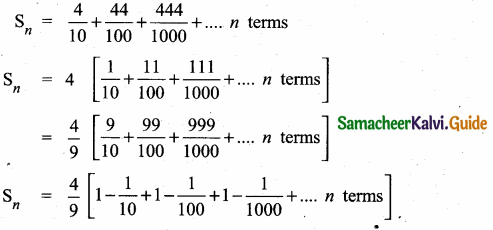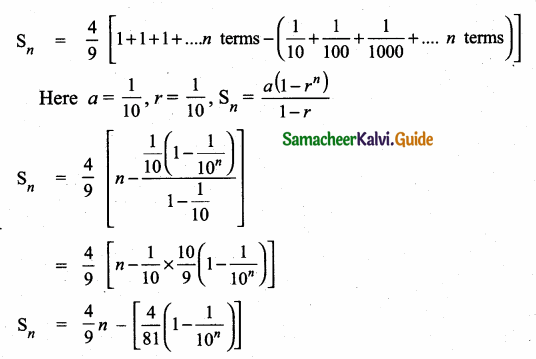(ii) 3 + 33 + 333 + ………… to n terms
Sn = 3 + 33 + 333 + …. to n terms
= 3[1 + 11 + 111 + …. to n terms]
= $$\frac { 3 }{ 9 }$$ [9 + 99 + 999 + …. n terms]
= $$\frac { 1 }{ 3 }$$ [(10 – 1) + (100 – 1) + (1000 – 1) + …… n terms]
= $$\frac { 1 }{ 3 }$$ [10 + 100 + 1000 + ….. n terms – (1 + 1 + 1 ….. n terms)]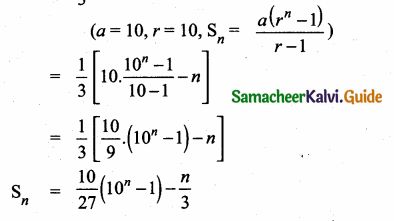Question 7.
Find the sum of the Geometric series 3 + 6 + 12 + …….. + 1536
3 + 6 + 12 …. +1536
a = 3, r = $$\frac { 6 }{ 3 }$$ = 2
tn = 1536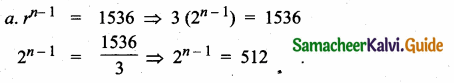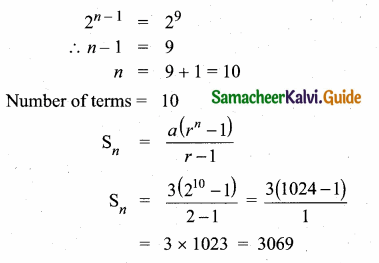∴ Sum of the series is 3069Question 8.
Kumar writes a letter to four of his friends. He asks each one of them to copy the letter and mail to four different persons with the instruction that they continue the process similarly. Assuming that the process is unaltered and it costs ₹2 to mail ong letter, find the amount spent on postage when 8th set of letters is mailed.
When kumar writes a letter to his friend.Friend writes a letter to another person.
It form a G.P
The G.P is 4, 16, 64,………
Here a = 4, r = 4
The last term is 4 (4)8-1 = 4(4)7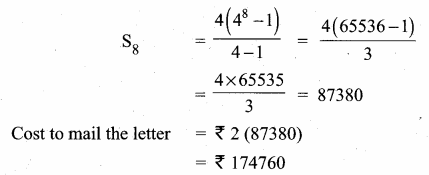Question 9.
Find the rational form of the number 0.123 .
Let x = $$\overline { 0.123 }$$
= 0.123123123….
= 0.123 + 0.000123 + 000000123 + ….
This is an infinite G.P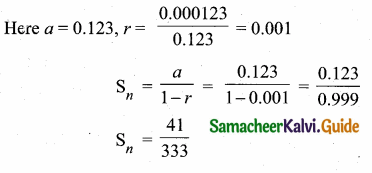Question 10.
If Sn = (x + y) + (x2 + xy + y2) + (x3 + x2y + xy2 + y3) + ………… n terms then prove that
Sn = (x + y) + (x2 + xy + y2) + (x3 + x2y + xy2 + y3) + …….. n
Multiply by x
x Sn = x(x + y) + x(x2 + xy + y2) + x(x3 + x2y + xy2 + y3) + ……….. n
= x2 + xy + x3 + x2y + xy2 + x4 + x3y + x2y2 + xy3 + …… n terms ……(1)
Multiply by y
ySn = y(x + y) + y(x2 + xy + y2) + y(x3 + x2y + xy2 + y3) + ….. n
= xy + y2 + x2y + xy2 + y3 + x3y + x2y2 + xy3 + y4 + ….. n terms
Subtract (1) and (2)
x Sn – y Sn = x2 + xy + x3 + x2y + xy2 + x4 + x3y + x2y2 + xy3 + …….
– xy + y2 + x2y + xy2 + y3 + x3y + x2y2 + xy3 + y4 + ……
(x – y) Sn = (x2 + x3 + x4 + ……) – (y2 + y3 + y4 + ……)
[ a = x2; r = x and a = y2; r = y, Sn = $$\frac{a\left(r^{n}-1\right)}{r-1}$$]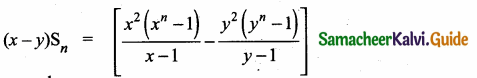Hence it is proved.